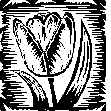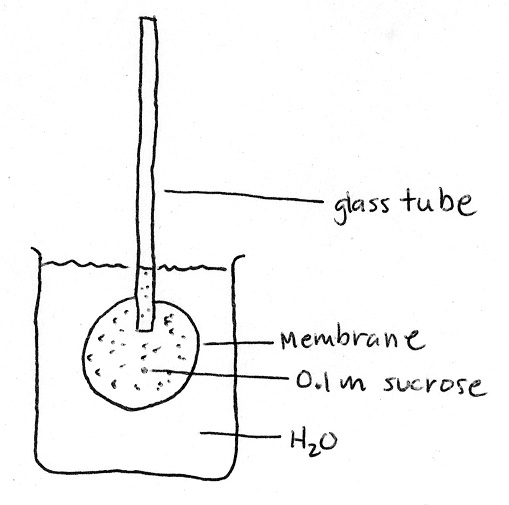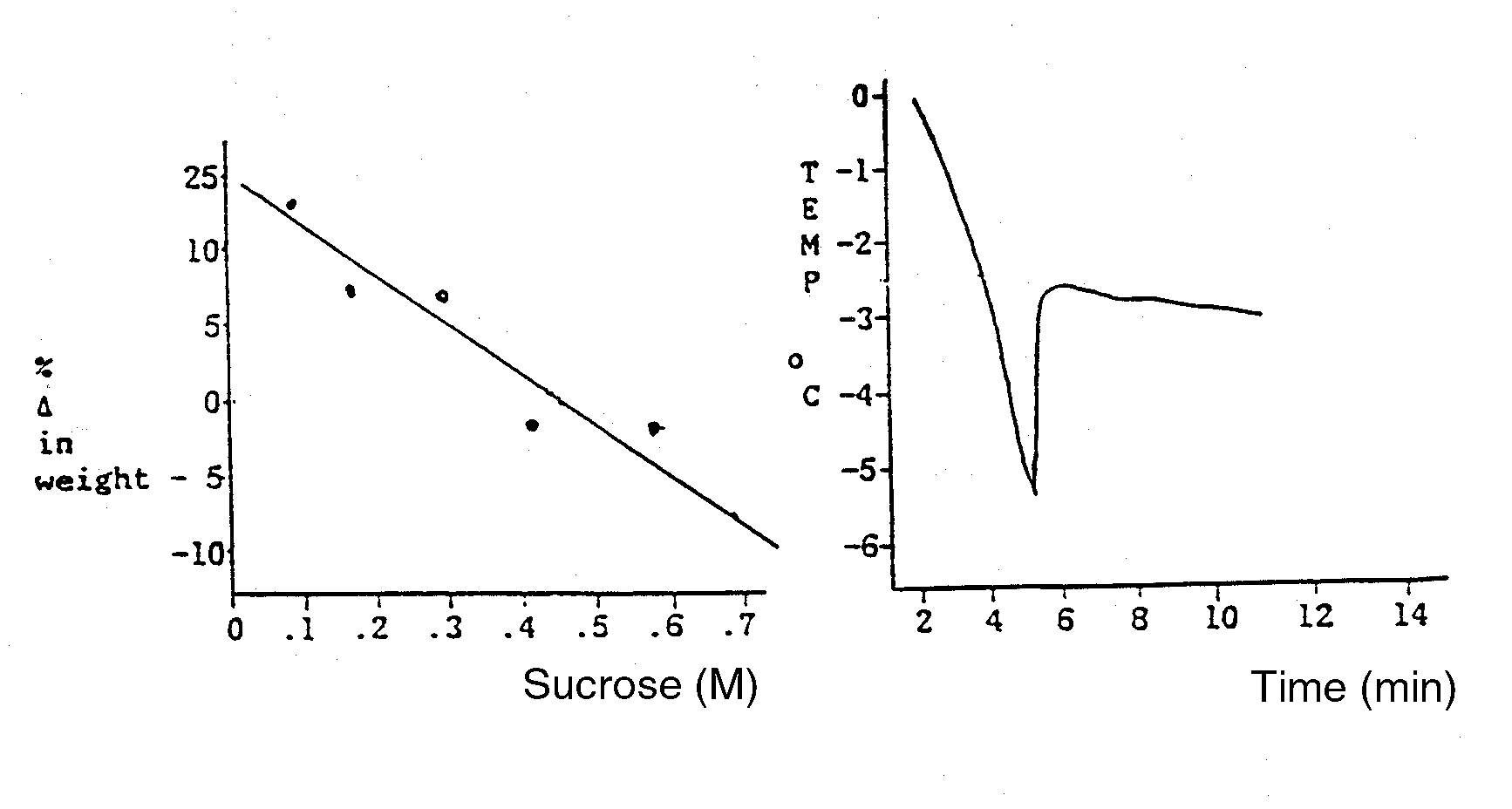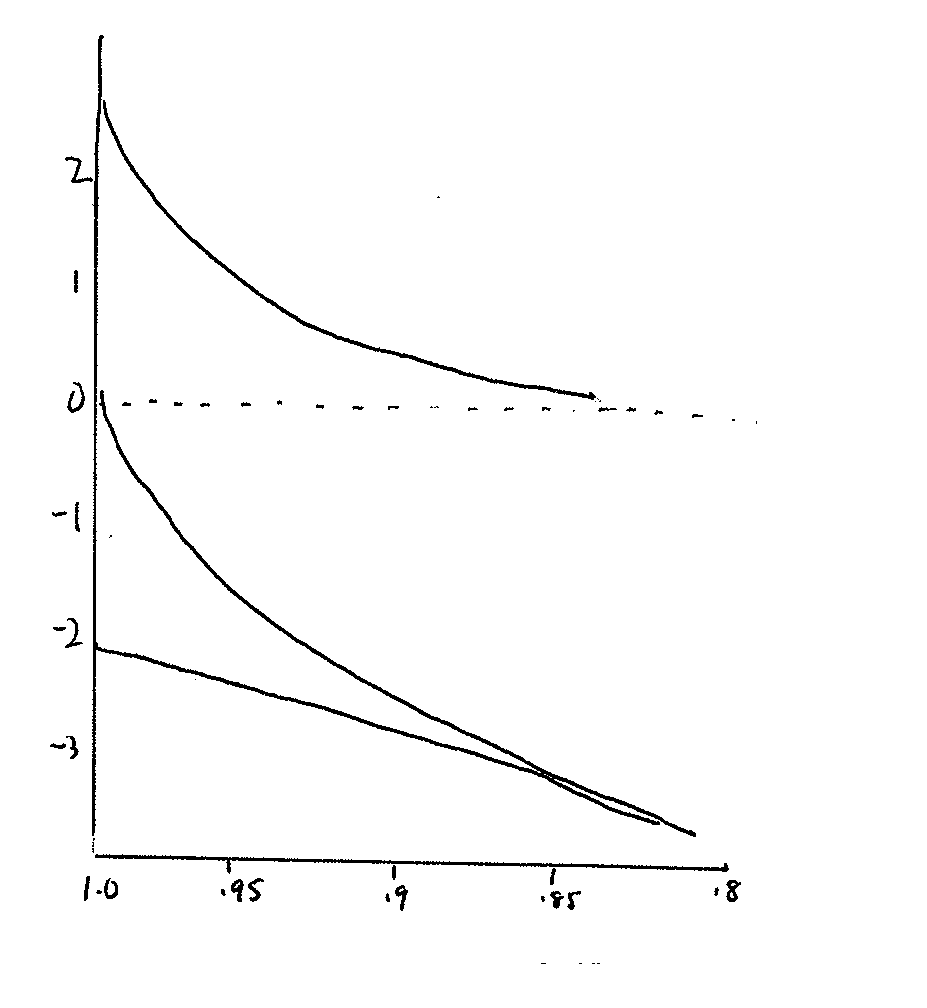Plant Physiology (Biology 327)  - Dr. Stephen G. Saupe;  College of St. Benedict/ St. John's University;  Biology Department; Collegeville, MN  56321; (320) 363 - 2782; (320) 363 - 3202, fax;    ssaupe@csbsju.edu

Diffusion, Osmosis & Water Potential Quiz

1. What is the water potential (Ψw) of a beaker of pure water ___. Pressure potential?  __ Solute potential? ____.

2. Calculate the osmotic potential (Ψs) of a 2.4 molal sucrose solution at 24oC. Show your work.

3. Calculate the water potential (Ψw) of a 2.4 molal sucrose solution at 24oC. Show your work.

4. Assume that a plant cell with a water potential of -1.0 MPa is placed in a beaker containing a sucrose solution that has a water potential of -4.0 MPa. Further, assume the temperature of the solution is 24 C. Thus:
 a. The plant cell will become: a. larger     b. smaller   c. not change b. The weight of the plant cell will: a. increase   b. decrease   c. not change c. The concentration of the sucrose solution in the beaker will: a. increase   b. decrease  c. not change d. The turgidity of the plant cell will: a. increase   b. decrease  c. not change e. The osmotic potential of the sucrose solution will become: a. more negative  b.  less negative f. There will be a net movement of water from the: a. cell to the solution  b. solution to the cell g. After a few hours the cell is removed. A drop of sucrose (-4.0 MPa) placed in the solution will: a. float    b. sink    c. hover & disperse h. The refractive index of the sucrose solution will: a. increase  b. decrease  c. not change i. The cell will likely plasmolyze: a. false  b. true j. The initial concentration of the sucrose solution (in molality) is: k. Assume that at equilibrium, the water potential of the cell becomes -3.5 MPa. Thus, the concentration of the sucrose solution (in molality) in the beaker at equilibrium is:

5. Calculate the pressure (MPa) in a cell if Ψw = -1.7 MPa and Ψs = -4.2 MPa

6. If Ψs for a tissue is -0.80 MPa, what do you predict the freezing point depression of expressed sap will be? Show your work.

Osmometer QuestionThe diagram represents a simple osmometer.  The membrane is freely permeable to water by not solute (sucrose).  The membrane is inelastic.  Inside the membrane is a solution of 0.1 molar sucrose.

 There will be a net movement of water ________ the membrane sac.         a.  into        b.  out of The water level in the tube will:         a.  fall        b.  rise        c.  not change The pressure in the sac will:         a.  decrease    b. increase        c.  not change Sketch a graph plotting water potential in the sac vs. time Sketch a graph plotting osmotic potential in the sac vs. time Sketch a graph plotting pressure in the sac vs. time.Some Multiple Choice Questions
: (circle all answers that apply)

1.  A cell whose internal osmotic concentration is 0.3 osmoles/liter is placed in a solution that is 0.5 osmoles/liter.  The solution is:

a.  Isoosmotic to the cell            d.  Isotonic to the cell
b.  Hypoosmotic to the cell       e.  Hypertonic to the cell
c.  Hyperosmotic to the cell      f.  Hypotonic to the cell

2.   A cell is placed in a solution and swells.  The solution is:

a.  Isoosmotic to the cell            d.  Isotonic to the cell
b.  Hypoosmotic to the cell       e.  Hypertonic to the cell
c.  Hyperosmotic to the cell      f.  Hypotonic to the cell

3.  A cell is placed in a solution and the cell swells.  The solution is probably, but not necessarily:
a.  isoosmotic to the cell
b.  hypoosmotic to the cell
c.  hyperosmotic to the cell.

Using water potential terminology, explain why
:

1. Over-fertilizing your lawn will cause it to �burn� (the leaves wilt, become dry and fall off).

2. Strawberries become juicy when you add sugar to them.

3. Cooks put wilted lettuce in cold water.

Calculation Question:

Determine the water potential of a cell if Ψp = 0.3 MPa and Ψs = -0.5 MPa. Calculate the pressure in a cell if Ψw = -0.1 and Ψs = -0.2?   Calculate the osmotic potential of a cell (Ψs) if  Ψw = -0.1 and Ψp = 0.8.

Measuring Water Potential - A Simulation

A plant physiologist wanted to study the water relations of a turnip. She prepared cores from the turnip with a core borer and then determined their change in weight after incubation in a graded series of sucrose solutions at 25 C.  These data are presented in Fig 1. She also ran a freezing point depression experiment on the turnip sap and these data are also plotted in Fig 2. Using these data, answer the following questions:

1. What is the water potential (MPa) of the turnip cells (show your work)?

2. What is the osmotic potential (MPa) of the turnip cells (show your work)?

3. What is the pressure potential (MPa) of the turnip cells (show your work)?Fig 1.  Gravimetric Data Fig 2.  Freezing Point Data

Hofler Diagram Question:   The sketch below represents a Hofler diagram.  Use it to answer the following questions.

1. Which of the following is the appropriate label for the "X" axis?
a.  Water, pressure or solute potential (Ψ, MPa)
b.  Relative cell volume (
Δ V/V)

2. Which is the appropriate label for the Y axis?
a.  Water, pressure or solute potential (Ψ, MPa)
b.  Relative cell volume (
Δ V/V)

3. Write the labels on the graph.

4. Which line best reflects the change in water potential that would occur as a cell volume changes?  Label this line Ψw

5. Which line best reflects the change in pressure potential that would occur as a cell volume changes?  Label this line Ψp.

6. Which line best reflects the change in solute potential that would occur as a cell volume changes?  Label this line Ψs

7. What do you conclude from this graph?Diffusion Coefficient Question:  To calculate the time it takes for a substance to diffuse a particular distance, use the equation time (s) = L2/Ds where L is the diffusion length (in meters) and Ds is the diffusion coefficient.  For glucose, Ds = 10-9m2s-1.

1. Calculate the time it will take a glucose molecule to diffuse through a single cell wall if the diameter of the wall is 100 �m.

2. Calculate the time it would take a glucose molecule to diffuse 1.0 meter.

 | Top | SGS Home | CSB/SJU Home | Biology Dept | Biol 327 Home | Disclaimer |

Last updated:  02/24/2009     � Copyright  by SG Saupe